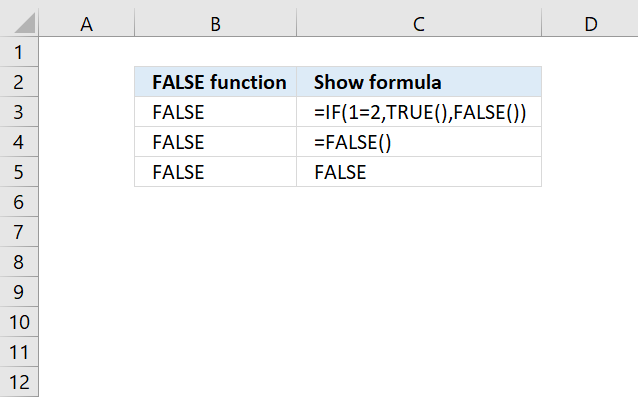Author: Oscar Cronquist Article last updated on May 04, 2022The FALSE function returns the logical (boolean) value FALSE.

=FALSE()

## 2. FALSE Function Arguments

The FALSE function has no arguments.

## 3. FALSE Function ExampleExcel interprets the boolean value FALSE exactly the same as =FALSE(), you most often don't need the FALSE function. It exists primarily for compatibility with other software.

Formula in cell C3:

=IF(1=2, TRUE(), FALSE())

### Explaining formula

#### Step 1 - Logical expression

The equal sign lets you compare value to another value.

1=2

returns FALSE.

#### Step 2 - Evaluate IF Function

The IF function returns one value if the logical test is TRUE and another value if the logical test is FALSE.

IF(logical_test, [value_if_true], [value_if_false])

IF(1=2, TRUE(), FALSE())

becomes

IF(FALSE, TRUE(), FALSE())

and returns FALSE(). FALSE() returns FALSE.